Home > A2C > Chapter 7 > Lesson 7.1.1 > Problem7-10

7-10.
1. Each cube below is 1 cm on a side. Homework Help ✎

1.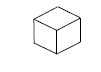2.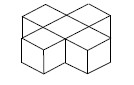3. ?

4.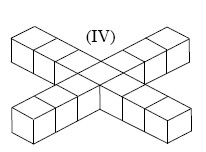1. Based on the pattern, find the volume of Figure III.

2. If the pattern continues, write an expression to represent the volume of figure N. What kind of sequence is this?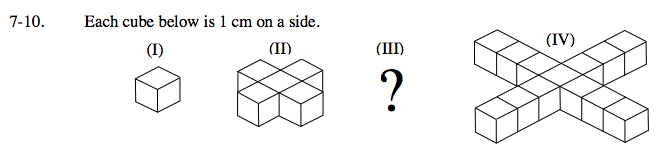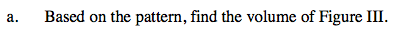What is the difference between Figure (II) and Figure (IV)?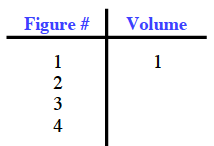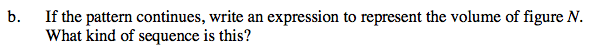Find the common difference between the volumes of each figure.

4n − 3, arithmetic